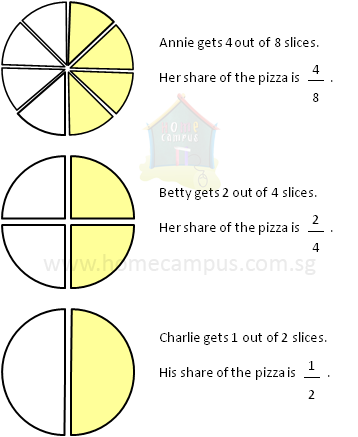## Equivalent Fractions, Simplifying Fractions, GCF

Practice Unlimited Questions

#### 2. What are Equivalent Fractions?

Fractions that are equal to each other are called equivalent fractions.

Example:It is clear from the images above that the yellow coloured portions of the 3 pizzas are equal to each other.
So,
 4 8
=
 2 4
=
 1 2

Therefore, Annie, Betty and Charlie each got
 1 2
a pizza.

When possible, we should simplify fractions.

#### 3. Why should we simplify fractions?

We should simplify fractions because simplified fractions are easier to understand.

Example:
So, though
 51 85
is the same as
 3 5
, it is much easier to understand
 3 5
than
 51 85
.

#### 4. Simplify the fraction    4 8 .

To simplify the fraction, keep dividing the numerator and the denominator by a common factor until you can't divide them any further.

So, to simplify the fraction
 4 8
, divide both 4 and 8 by 2 (as 2 is a common factor of 4 and 8).
 4 / 2 8 / 2
=
 2 4

2 and 4 can be divided further by 2.

 2 / 2 4 / 2
=
 1 2

 1 2
is the simplest form of the fraction
 4 8
.

#### 5. Simplify the fraction    180 240 .

To simplify the fraction
 180 240
, divide both 180 and 240 by their common factors.

It is easy to see that both numbers can be divided by 10.

 180 / 10 240 / 10
=
 18 24

18 and 24 can be divided further by 2.

 18 / 2 24 / 2
=
 9 12

9 and 12 can be divided further by 3.

 9 / 3 12 / 3
=
 3 4

 3 4
is the simplest form of the fraction
 180 240
.